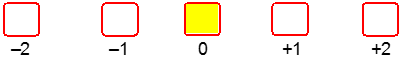Clutch Prep is now a part of Pearson
Ch.7 - Quantum MechanicsWorksheetSee all chapters

# Quantum Numbers: Magnetic Quantum Number

See all sections
Sections
Wavelength and Frequency
Speed of Light
The Energy of Light
Electromagnetic Spectrum
Photoelectric Effect
De Broglie Wavelength
Heisenberg Uncertainty Principle
Bohr Model
Emission Spectrum
Bohr Equation
Introduction to Quantum Mechanics
Quantum Numbers: Principal Quantum Number
Quantum Numbers: Angular Momentum Quantum Number
Quantum Numbers: Magnetic Quantum Number
Quantum Numbers: Spin Quantum Number
Quantum Numbers: Number of Electrons
Quantum Numbers: Nodes

The Magnetic Quantum Number gives the location of an electron within a specific orbital.

###### Magnetic Quantum Number

Concept #1: Magnetic Quantum Number

Example #1: Provide the identity of a set of orbitals that exist in the 4th principal level and f sublevel

Concept #2: The Magnetic Quantum Number (ml)

Example #2: Which of the following is not a valid magnetic quantum number for the 7f set of orbitals?

Practice: How many different values of ml are possible for a 4d set of orbitals?

Practice: Select a correct set of values for an electron found within the designated 5f orbital.Practice: Which of the following statements is false?

a) A set of d orbitals contains 5 orbitals.

b) A set of 4s orbitals would have more energy than a set of 3p orbitals.

c) The second shell of an atom possesses d orbitals.

d) A set of f orbitals contains 3 orbitals.

e) The first energy level contains only s orbitals.